International Journal of Energy and Power Engineering
Volume 5, Issue 2-1, March 2016, Pages: 1-8

Assessing the Impact of Load and Renewable Energies’ Uncertainty on a Hybrid System

Amin Shokri Gazafroudi

EE Department, Imam Khomeini International University, Qazvin, Iran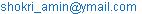Amin Shokri Gazafroudi. Assessing the Impact of Load and Renewable Energies’ Uncertainty on a Hybrid System. International Journal of Energy and Power Engineering. Special Issue: Electricity Market. Vol. 5, No. 2-2, 2016, pp. 1-8. doi: 10.11648/j.ijepe.s.2016050202.11

Abstract: As increasing of fossil fuels and the trend of expiring, using of alternative fuels has been on the agenda of most countries particularly in the past two decades. In the meantime, the using of wind energy and solar radiation are extremely popular as sources of green energy and high-efficiency. Hence, the prediction of wind and solar power is important. The power output of these power plants depends on wind speed, temperature and radiation. In this paper, the uncertainty of wind and solar power generation, and load forecasting are considered based on correlation analysis on wind power, solar radiation, and ambient temperature time series. Predicted values are given to the hybrid system (wind–fuel cell–photovoltaic) to provide electrical load for the 24-hours. Finally, the proposed model is applied to demonstrate its effectiveness based on actual examples information of load, wind, radiation, temperature of wind farm and solar power plants.

Keywords: Renewable Energies, Neural Network, Correlation Analysis, Forecasting Method, Uncertainty

1. Introduction

Increasing human population has direct effect on electrical energy demand which has increased the electrical power generation . So that in 2007, 26 % of pollution and greenhouse gas emissions are resulted from the production of electricity and heat . Therefore in recent years, electricity generation based on renewable energies, has been highly regarded in different countries . Electrical power generation based on wind energy, has been fastest growing among the renewable energy sources . This is due to its environmental benefits. It is estimated that in 2020, 12% of the world's electricity will supply from wind power .So, wind power generation will play an important role in electricity supply. Inability of wind power to follow the load pattern is important point and required to design a hybrid system in are as separated from the main system. In this paper, a hybrid system (wind-fuel cell– photovoltaic) is designed to provide the load in a day. So, the radiation and temperature forecasts which are solar system inputs. They are as important as wind power forecasting.

An accurate forecasting of the electrical load, wind speed, solar radiation and temperature can have positive impacts on the production scheduling. In this paper, short-term prediction of load, wind speed, solar radiation and ambient temperature are applied by feed forward neural network according to their historical time series. Finally, the forecasted data are given in to a hybrid system. The proposed model is shown in Fig.1.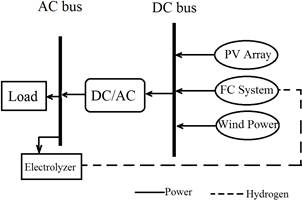Fig. 1. System Schema.

Different sections of this paper are divided as follows. In Section 2, load model is presented. In Section 3, radiation, temperature and wind speed are forecasted. In section 4, designing and simulating the Solar panels, fuel cell and wind power plants are presented. Finally, the conclusion drawn from proposed hybrid system is provided in section 6.

The 24 hour load curve is shown in Fig. 2. According to the load curve, load has two peaks. One of them is in the middle of day and related to add cooling device. The second one is at early evening and related to add lighting. The actual load curve in comparison with predicted load curve in 24-hourand a week period are shown in Fig. 2 and Fig. 3.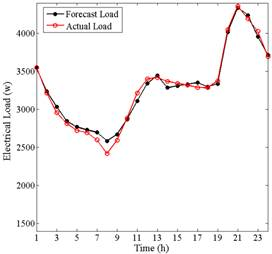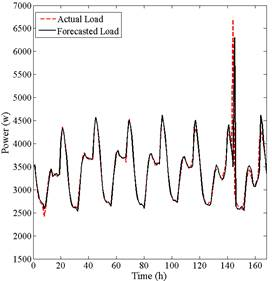Fig. 3. A week load forecast.

3. Wind Speed, Radiation and Temperature Forecast

In this section, artificial neural networks and correlation analysis are presented as methods for cognitive behavior of wind speed, radiation and temperature data, and the predicted data are resulted by a forecast engine based on artificial neural network. Wind speed, radiation and temperature time series are shown respectively in Fig. 4, Fig. 5 and Fig. 6.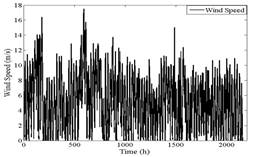Fig. 4. Wind speed time series.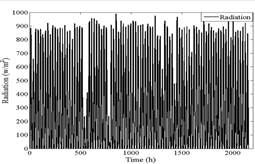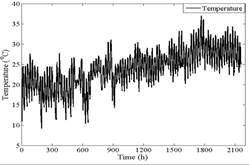Fig. 6. Temperature time series.

3.1. Forecasting Method

Neural network is the nonlinear technique that can be applied to detect patterns and find the complex behavior of a system through its input and output data. A neural network (NN) needs learning to find the complex behaviors of data. Despite the NN is simple, but it is a powerful and flexible tool to forecast. NN have an ideal output by choosing appropriate data number for training, inputs, hidden layers and neurons in each layer. NN can approximate any nonlinear function . Thus, depending on how much the system inputs are appropriate; we will expect a better response of system outputs.

Before modeling a system, it is necessary to find the relation between the measured parameters. The correlation coefficient matrix is a normalized value that expresses the strength of linear relationship between two variables. Correlation coefficient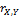between two random variables X and Y, with mean valuesand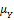and standard deviation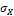and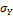, expresses covariance between normalized variables. The correlation coefficient obtained from the following equation: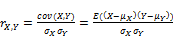(1)

The appropriate inputs of the neural network are selected by taking the minimal value of the correlation coefficient.

3.2. Wind Speed Forecasting

Wind power is proportional to air density, wind speed and environmental obstacles . Air density is an exponential function of air temperature and pressure. So wind power is dependent on weather conditions such as wind speed, wind direction and temperature. Also, the wind power has great relationship with its past values in any moment .Wind power is a non-linear function of wind parameters such as wind speed, air density and turbulent kinetic energy . Wind power forecasting methods can be divided into physical models and time series or statistical models .

In physical model, wind speed is estimated in are going under the physical laws governing atmosphere, its behavior and the corresponding from wind power in a specified level of wind farm . These models consider the physical characteristics of the environment, orography, atmospheric conditions (such as pressure and temperature) to estimate the future wind speed and wind power .

In statistical model tries to predict the future wind’s speed and power by finding the relationship between the parameters of the historical data. In this paper, the statistical model is applied to forecast the short-term wind speed. Wind speed autocorrelation curve is shown in Fig. 7 and the correlation between the wind speed and radiation is shown in Fig. 8.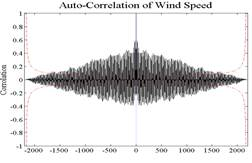Fig. 7. Wind speed autocorrelation curve.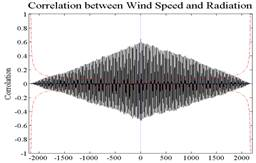Fig. 8. Correlation between the wind speed and radiation.

The selected appropriate inputs related to wind speed depending on the amount of their cross-correlation which presented in Tables 1.

Table 1. Selected inputs for W(t).

 Rank Selected Inputs Cross-Correlation Rank Selected Inputs Cross-Correlation 1 W(t-1) 0.847 3 S(t-1) 0.637 2 W(t-2) 0.718 4 S(t-2) 0.615

In Table 1,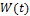represents the wind speed and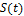represents the solar radiation. As indicated in Table 1, wind speed at any moment has the highest correlation with wind speed in an hour ago, wind speed in two hours ago, solar radiation in an hour ago and solar radiation in two hours ago. Despite the correlation analysis and find the correlation between wind speed and temperature, the temperature isn’t found as an input in Table 1. This is due to the highest correlation between wind speed and temperature is 0.336, which is not the useful input for wind speed forecast. The actual wind speed curve in comparison with predicted wind speed curve in 24-hour, and a week period are shown in Fig.9 and Fig. 10.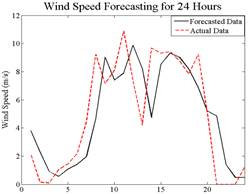Fig. 9. 24-hour wind speed forecast.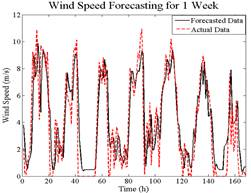Fig. 10. A week wind speed forecast.

The appropriate inputs for solar radiation forecasting are selected by correlation analysis as wind speed prediction. Those data are selected as inputs for solar radiation forecasting engine which their cross-correlation are more than 0.85.The selected appropriate inputs related to solar radiation depending on the amount of their cross-correlation are presented in Tables 2.

Table 2. Selected inputs for S(t).

 Rank Selected Inputs Cross-Correlation Rank Selected Inputs Cross-Correlation 1 S(t-1) 0.909 3 S(t-48) 0.864 2 S(t-24) 0.882 4 S(t-72) 0.852

As indicated in Table 2, solar radiation at any moment has the highest correlation with solar radiation in an hour ago, solar radiation in 24 hours ago, solar radiation in 48 hours ago and solar radiation in 72 hours ago. Periodic behavior of radiation is clear from the Fig. 12.

Despite the correlation analysis and find the correlation between solar radiation and temperature, the temperature isn’t found as an input in the Table 2. This is due to the highest correlation between solar radiation and temperature is 0.426, which is not the useful input for solar radiation forecast. The actual solar radiation curve in comparison with predicted solar radiation curve in 24-hour and a week period are shown in Fig. 11 and Fig. 12.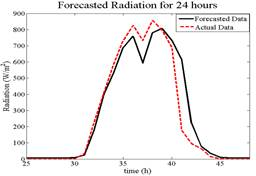Fig. 11. 24-hour solar radiation forecast.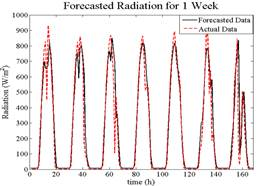Fig. 12. A week solar radiation forecast.

3.4. Temperature Forecasting

The appropriate inputs for temperature forecasting are selected by correlation analysis. Those data are selected as inputs for temperature forecasting engine which their cross-correlation are more than 0.85. The selected appropriate inputs related to temperature depending on the amount of their cross-correlation are presented in Tables 3.

Table 3. Selected inputs for T(t).

 Rank Selected Inputs Cross-Correlation Rank Selected Inputs Cross-Correlation 1 T(t-1) 0.972 3 T(t-23) 0.858 2 T(t-2) 0.914 4 T(t-24) 0.867

In Table 3,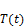represents the temperature. As indicated in Table3, temperature at any moment has the highest correlation with temperature in an hour ago, temperature in 2 hours ago, temperature in 23 hours ago and temperature in 24 hours ago. This correlation indicates that the temperature has a periodic behavior such as solar radiation. The actual temperature curve in comparison with predicted temperature curve in 24-hour and a week period are shown in Fig. 13 and Fig. 14.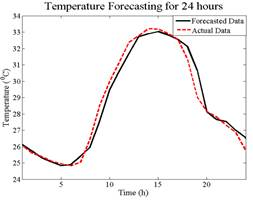Fig. 13. 24-hour temperature forecast.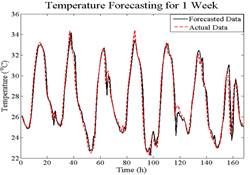Fig. 14. A week temperature forecast.

4. Designing and Simulating the Hybrid System

4.1. Wind Power Plants Simulation

Analysis of electric energy and the reliability benefits needed to accurately simulation of winds peed for each specific site. Wind speed time series can be modeled with many distributions such as Weibull and normal distributions. Assuming a normal distribution is used to describe the wind speed data particular site, the normal distribution density function is: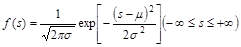(2)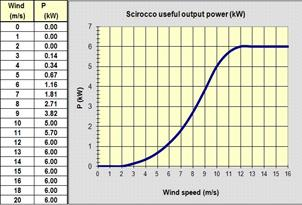Fig. 15. Power curve of a wind turbine.

Wind speed can be approximated by the following equation :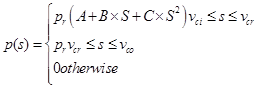(2)

Where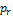is rated power capacity,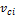is cut-in speed and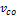is cut-out speed. The parameters A, B and C are given by Eq. (4):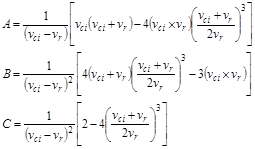(3)

In section 3-2, we forecast wind speed for a 24-hour period. According to Eq. (3) and parameters A, B and C, forecasted wind power and actual wind power are shown in Fig. 16.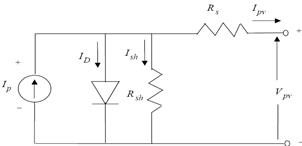Fig. 16. Electrical model of the PV array.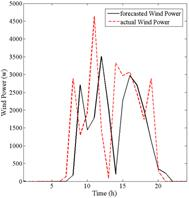Fig. 17. 24-hour power output of wind power plant.

As shown in Fig. 16, when the wind speed is lower than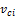and upper than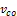, output of wind power plant is zero.

4.2. Solar Arrays Simulation

PV arrays can be simulated by an electrical circuit (Fig.17), which includes a current source and a diode. Current power supply circuit is representative of solar radiation in this circuit .

Current is fixed to a value of voltage array, after that with voltage increasing, the diode opened and the output current is reduced drastically . Output voltage and current are obtained from the reference  equations. Simulation is performed by considering the values which mentioned in  and , the MA 36/45solararraysresultsis mentioned finally according to .

Table 4. Electrical information for a MA36/45 PV array .

 Maximum Power 45 W No Load Voltage 205 volt Voltage in Maximum Power 16.7 volt Short Circuit Current 2.96 volt Current in Maximum Power 2.74 volt

According to the system load, series and parallel models of solar panels are shown in Fig. 18. Forecasted solar power and actual solar power are shown in Fig. 19.

4.3. Fuel Cells Simulation

As the clean energy conversion technology, fuel cell is the promising alternative to electrical power generation . In this paper, PEM fuel cell is simulated because of the quick Startup and high efficiency and power-voltage characteristic has been evaluated based on  simulations.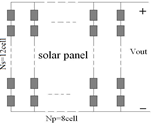Fig. 18. Series and parallel models of solar panels.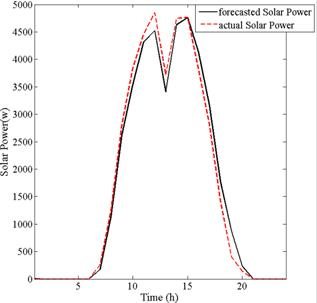Fig. 19. 24-hour power output of solar power plant.

4.4. Simulation Results

In this section, the impact of uncertainty of load forecasting, wind, radiation and temperature are investigated on system. Hence, the system will be discussed in the four cases:

As shown in Fig. 21, power used in the fuel cell is not significantly different between the four cases and it is because of accurate load forecasting. Note that the wind and radiation don’t work in this situation. So the uncertainty in load forecast has little impact on the system.

Electrolyzer power curves are shown in Fig. 22. Due to suitable gust of wind and high solar radiation during the day, system power generation exceeds the load and the extra Power generation spend to product hydrogen and stores it in the hydrogen tank. As shown in Fig. 22, uncertainties have a large impact on the electrolyzer power. Since, electrolyzer power affected by wind power and wind forecast has high uncertainty. Therefore, cases B and D are very different. Cases A and C are so similar and it’s also due to involved the uncertainties of wind speed and radiation forecasts in system.

The amount of hydrogen stored in a tank is shown in Fig. 23. Case C and A are similar. Cases B and D are very different, due to wind uncertainty. Whatever the wind uncertainty is greater, the incorrect scheduling and consequently more fuel consumption and costs will suffer.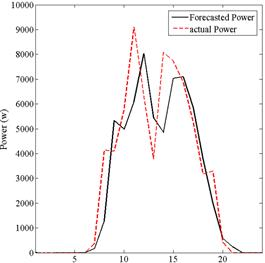Fig. 20. 24-hour total power generation by wind and solar.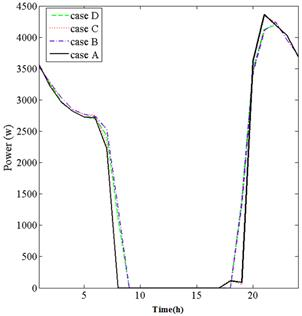Fig. 21. 24-hour fuel cell output power.

5. Conclusion

With hybrid industrial development in the field of renewable energies, using hybrid energies (wind-fuel cell– photovoltaic) in remote or off-grid areas is deemed important and profitable. Hence, the accurate predictions of wind speed, radiation and temperature are important. We can do the accurate scheduling of generation, fuel and cost by considering the exact short-term and long-term of load, wind, radiation and temperature forecasting.

In this paper, a hybrid system (Wind-FC-PV) is simulated to supply the variable load, which wind speed, radiation and load are system inputs. According to the forecasting results and hybrid systems outputs, wind speed uncertainty is more than the radiation and temperature uncertainties. Thus, wind forecast plays an important role in hybrid systems.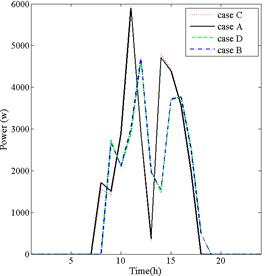Fig. 22. 24-hour electrolyzer power.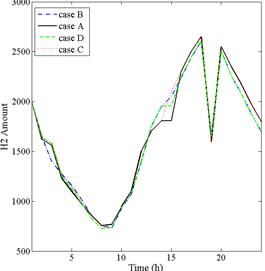Fig. 23. 24-hour hydrogen tank.

References

1. Johnson PL, Negnevitsky M, Muttaqi KM. "Short term wind power forecasting using adaptive neuro-fuzzy inference systems", Australasian Universities Power Engineering Conference, AUPEC 2007, Dec. 2007, pp. 1–6.
2. A report prepared by European Environment Agency (EEA). Greenhouse gas emission trends and projections in Europe 2009 tracking progress towards Kyoto targets, Report No. 9/2009. Available: [online] www.eea.europa.eu
3. Ortega-Vazquez MA, Kirschen DS. "Estimating the spinning reserve requirements in systems with significant wind power generation penetration". IEEE Transactions on Power Systems 2009; 24(1):114–124.
4. N. Amjady, F. Keynia, and H. R. Zareipour. "A new hybrid iterative method for short-term wind speed forecasting". Euro. Trans. Elect. Power, DOI: 10.1002/etep.463.
5. N. Bigdeli, K. Afshar, Amin Shokri Gazafroudi, M. Yousefi Ramandi, "A comparative study of optimal hybrid methods for wind power prediction in wind farm of Alberta, Canada", Renewable and Sustainable Energy Reviews, Volume 27, November 2013, Pages 20-29.
6. S. Fan, J. R. Liao, R. Yokoyama, L. Chen, and W.-J. Lee, "Forecasting the wind generation using a two-stage network based on meteorological information", IEEE Trans. Energy Convers., vol. 24, no. 2, pp. 474482, Jun. 2009.
7. Y. K. Wu and J. S. Hong, "A literature review of wind forecasting technology in the world", in Proc. IEEE Power Tech. Conf., Jul. 2007, pp. 504509.
8. F. Ö. Thordarson, H. Madsen, H. A. Nielsen, and P. Pinson," Conditional weighted combination of wind power forecasts", Wind Energy, vol. 13, no. 8, pp. 751763, Nov. 2010.
9. G. Sideratos and N. Hatziargyriou, "Using radial basis neural networks to estimate wind power production", in IEEE Power Engineering Society General Meeting, 2007, DOI: 10.1109/PES.2007.385812.
10. G. Giebel, The State-Of-The-Art in Short-Term Prediction of Wind Power a Literature Overview 2003 [Online]. Available: http://anemos.cma.fr/download/ANEMOS_D1.1_StateOfTheArt_v1.1.pdf
11. I. J. Ramirez-Rosado, L. A. Fernandez-Jimenez, C. Monteiro, J. Sousa, and R. Bessa, "Comparison of two new short-term wind-power forecasting systems", Renewable Energy, vol. 34, no. 7, pp. 18481854, Jul. 2009.
12. M. Lei, L. Shiyan, J. Chuanwen, L. Hongling, and Z. Yan, "A review on the forecasting of wind speed and generated power", Renewable Sustain. Energy Rev., vol. 13, no. 4, pp. 915920, May 2009.
13. L. Ma, S. Y. Luan, C. W. Jiang, H. L. Liu, and Y. Zhang, "A review on the forecasting of wind speed and generated power," Renew. Sust. Energy Rev., vol. 13, no. 4, pp. 915–920, May 2009.
14. R. J. Bessa, V. Miranda, and J. Gama, "Entropy and correntropy against minimum square error in offline and online three-day ahead wind power forecasting", IEEE Trans. Power Syst., vol. 24, no. 4, pp. 16571666, Nov. 2009.
15. Methaprayoon K, Yingvivatanapong C, Lee WJ, Liao JR. "An integration of ANN wind power estimation into unit commitment considering the forecasting uncertainty". IEEE Transactions on Industry Applications 2007; 43(6):1441–1448.
16. www.solacity.com/Scirocco.htm.
17. Billinton R, Chowdhury AA. "Incorporation of wind energy conversion systems in conventional generating capacity adequacy assessment" IEE Proc. Gener. Transm. Distrib. 1992;139:47e56.
18. Giorsetto P, Utsurogi KF. "Development of a new procedure for reliability modeling of wind turbine generators" IEEE Trans. Power App. Syst.1983;102:134.
19. M. Farhat and L. Sbita, "Advanced fuzzy MPPT control algorithm for photovoltaic systems", science Academy Transactions on Renewable Energy Systems Engineering and Technology , vol. 1, no. 1, pp. 29-36, March 2011.
20. N. Hidouri, L.sbita, "Water Photovoltaic Pumping System Based on DTC SPMSM Drives," Journal of Electric Engineering: Theory and Application, Vol.1, no. 2, pp. 111-119, 2010.
21. M.S. Benghanem, Saleh N. Alamri, "Modeling of photovoltaic module and experimental determination of serial resistance," Journal of Taibah University for Science (JTUSCI),Vol. 1, pp. 94-105, 2009.
22. PhD thesis, YUN TIAM TAN FEBRUARY 2004. "IMPACT ON THE POWER SYSTEM WITH A LARGE PENETRATION OF PHOTOVOLTAIC GENERATION". PhD Thesis, Department of Electrical Engineering and Electronics UMIST.
23. Hassan Moghbelli, Robert Vartanian "Implementation of the Movable Photovoltaic Array to Increase Output Power of the Solar Cells''.
24. Mohammad Sarvi, Mohammad Mehdi Kazeminasab, Masoud Safari Shal "A New Fuzzy Control Method for Maximum Power Point Tracking of PEMFC’s System " The 2nd Conference on Hydrogen and Fuel Cell K. N. Toosi University of Technology, 2012.

 Contents 1. 2. 3. 3.1. 3.2. 3.3. 3.4. 4. 4.1. 4.2. 4.3. 4.4. 5.
Article ToolsAbstractPDF(832K)